Games
Problems
Go Pro!

# Mathematics

Lesson Plans > Mathematics

# Featured Lesson Plans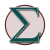How laziness can be a good teaching tool in a mathematics classroom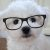142857 - Number theory, classroom projects, exploration, and activities surrounding an unusual number with mysterious properties.An alternate way of looking at the term 'term' and how we define it to our students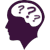Problems involving mixtures, including solutions, coins, populations - Algebra One Problems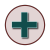Converting English language phrases to algebraic expressions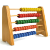A lesson that involves teaching students ways of using algebra to help them do arithmetic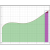Introducing students to the Fundamental Theorem of Calculus, using a bit of geometry to provide a basic proofAn overview of all the worksheets in the 'Mixed Practice' worksheet sets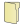# Full Directory Listing

10 ways to get wild about Math, 11 tips for building a strong Math foundation for kids, An Equation is not a Process, How kinesthetic folks learn Math, Mathematicians are Lazy, The Causes and Prevention of Math Anxiety, War Card Game - Modified for Subtraction PracticeLike us on Facebook to get updates about new resources